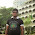## Objective Questions from Basic Electrical Engineering:

 In a 117Vrms utility circuit, the peak-to-peak voltage is approximately
A.  82.7 V
B.  165 V
C.  234 V
D.  331 V

 Watt hour is the unit of
A. Electric power
B. Electric capacity
C. Electric energy
D. Electric charge

 In India,electrical power is transmitted by
A. 1-phase ac system
B.  3-wire dc system
C.  3-phase 3-wire ac system
D. 2 wire dc system

 If two perfect sine waves have the same frequency and the same phase, the composite wave
A.  Is a sine wave with an amplitude equal to the difference between the amplitude of the waves
B.  Is a sine wave with an amplitude equal to the sum of the amplitudes of the two original waves
C.  Is not a sine wave, but has the same frequency as the two input waves
D.  Has zero amplitude (ie, it does not exist) because the two input waves cancel each other out.

 In a ac circuit the product of voltage and current is known as
A. Power
B. Real Power
C. Resistive power
D. Apparent power

 The advantage of AC over DC in utility applications is
A.  AC is easier to transform from one voltage to another
B.  AC is transmitted with lower loss in wires
C.  AC can be easily obtained from dc generators
D.  AC can be generated with less dangerous by products

 A network that does not have either voltage or current source is called ......network
A. Active
B. Passive
C. Resistive
D. Dummy

 The sixth harmonic of a ac wave whose period is 1 Milli second has a frequency of
A.  0.006Hz
B.  167.0 Hz
C.  7.0 kHz
D.  6 kHz

 A degree of phase represents
A.  6.28 cycles
B.  57.3 cycles
C.  1/60 of a cycle
D.  1/360 of a cycle

 A battery is source of
A. DC voltage
B. 1 phase AC voltage
C. 3 phase ac voltage
D. ac or dc voltage

6:31 PM

#### 1 comment:

1.